## ThinkDSP¶

This notebook contains code examples from Chapter 2: Harmonics

In :
# Get thinkdsp.py

import os

if not os.path.exists('thinkdsp.py'):
!wget https://github.com/AllenDowney/ThinkDSP/raw/master/code/thinkdsp.py


## Waveforms and harmonics¶

Create a triangle signal and plot a 3 period segment.

In :
from thinkdsp import TriangleSignal
from thinkdsp import decorate

signal = TriangleSignal(200)
duration = signal.period*3
segment = signal.make_wave(duration, framerate=10000)
segment.plot()
decorate(xlabel='Time (s)')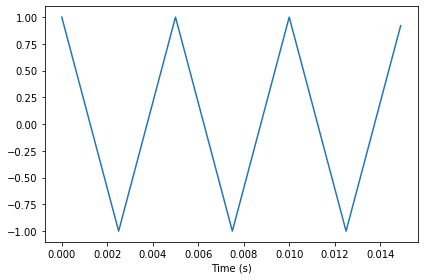Make a wave and play it.

In :
wave = signal.make_wave(duration=0.5, framerate=10000)
wave.apodize()
wave.make_audio()

Out:

Compute its spectrum and plot it.

In :
spectrum = wave.make_spectrum()
spectrum.plot()
decorate(xlabel='Frequency (Hz)')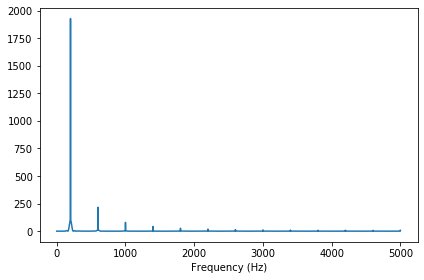Make a square signal and plot a 3 period segment.

In :
from thinkdsp import SquareSignal

signal = SquareSignal(200)
duration = signal.period*3
segment = signal.make_wave(duration, framerate=10000)
segment.plot()
decorate(xlabel='Time (s)')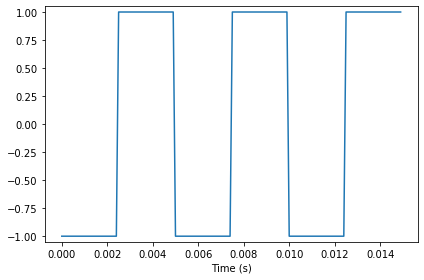Make a wave and play it.

In :
wave = signal.make_wave(duration=0.5, framerate=10000)
wave.apodize()
wave.make_audio()

Out:

Compute its spectrum and plot it.

In :
spectrum = wave.make_spectrum()
spectrum.plot()
decorate(xlabel='Frequency (Hz)')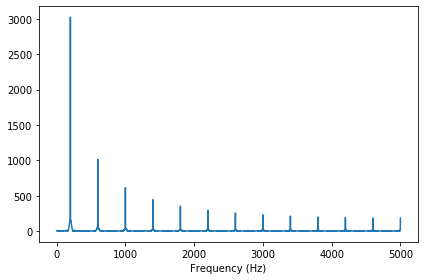Create a sawtooth signal and plot a 3 period segment.

In :
from thinkdsp import SawtoothSignal

signal = SawtoothSignal(200)
duration = signal.period*3
segment = signal.make_wave(duration, framerate=10000)
segment.plot()
decorate(xlabel='Time (s)')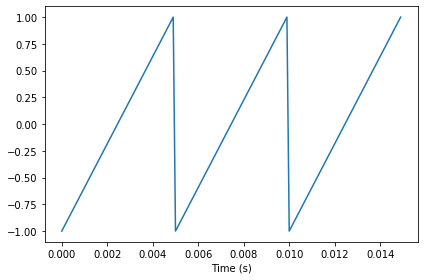Make a wave and play it.

In :
wave = signal.make_wave(duration=0.5, framerate=10000)
wave.apodize()
wave.make_audio()

Out:

Compute its spectrum and plot it.

In :
spectrum = wave.make_spectrum()
spectrum.plot()
decorate(xlabel='Frequency (Hz)')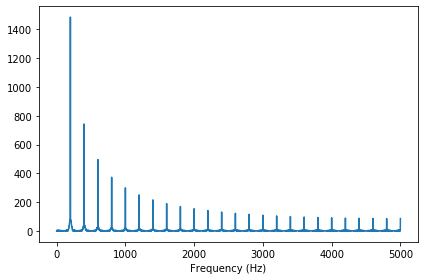### Aliasing¶

Make a cosine signal at 4500 Hz, make a wave at framerate 10 kHz, and plot 5 periods.

In :
from thinkdsp import CosSignal

signal = CosSignal(4500)
duration = signal.period*5
segment = signal.make_wave(duration, framerate=10000)
segment.plot()
decorate(xlabel='Time (s)')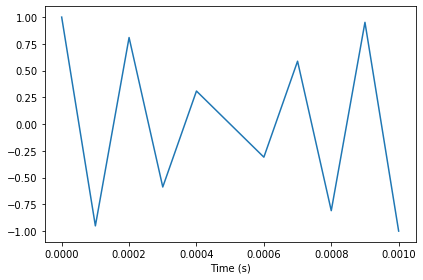Make a cosine signal at 5500 Hz, make a wave at framerate 10 kHz, and plot the same duration.

With framerate 10 kHz, the folding frequency is 5 kHz, so a 4500 Hz signal and a 5500 Hz signal look exactly the same.

In :
signal = CosSignal(5500)
segment = signal.make_wave(duration, framerate=10000)
segment.plot()
decorate(xlabel='Time (s)')Make a triangle signal and plot the spectrum. See how the harmonics get folded.

In :
signal = TriangleSignal(1100)
segment = signal.make_wave(duration=0.5, framerate=10000)
spectrum = segment.make_spectrum()
spectrum.plot()
decorate(xlabel='Frequency (Hz)')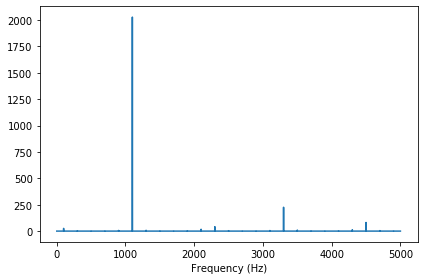## Amplitude and phase¶

Make a sawtooth wave.

In :
signal = SawtoothSignal(500)
wave = signal.make_wave(duration=1, framerate=10000)
segment = wave.segment(duration=0.005)
segment.plot()
decorate(xlabel='Time (s)')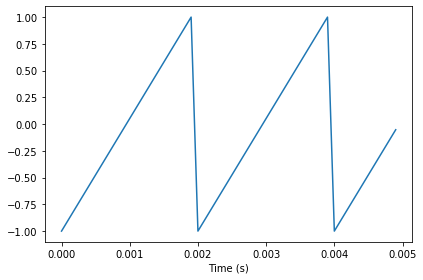Play it.

In :
wave.make_audio()

Out:

Extract the wave array and compute the real FFT (which is just an FFT optimized for real inputs).

In :
import numpy as np

hs = np.fft.rfft(wave.ys)
hs

Out:
array([ 5.11590770e-13+0.00000000e+00j,  2.19700679e-13-1.34559298e-13j,
-2.09548671e-13-6.74603523e-14j, ...,
4.19606174e-13+3.46000979e-14j, -5.63280756e-13+5.74915022e-14j,
-5.26315789e+02+0.00000000e+00j])

Compute the frequencies that match up with the elements of the FFT.

In :
n = len(wave.ys)                 # number of samples
d = 1 / wave.framerate           # time between samples
fs = np.fft.rfftfreq(n, d)
fs

Out:
array([0.000e+00, 1.000e+00, 2.000e+00, ..., 4.998e+03, 4.999e+03,
5.000e+03])

Plot the magnitudes vs the frequencies.

In :
import matplotlib.pyplot as plt

magnitude = np.absolute(hs)
plt.plot(fs, magnitude)
decorate(xlabel='Frequency (Hz)')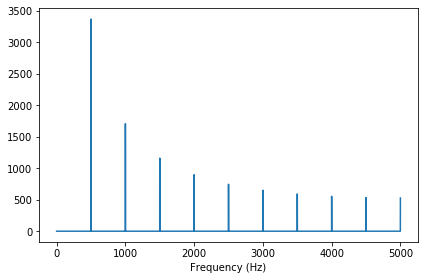Plot the phases vs the frequencies.

In :
angle = np.angle(hs)
plt.plot(fs, angle)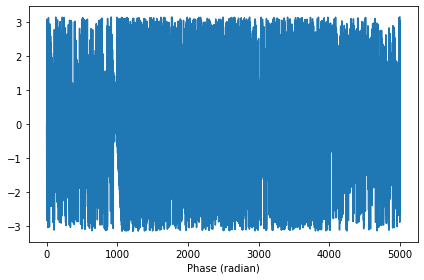## What does phase sound like?¶

Shuffle the phases.

In :
import random
random.shuffle(angle)
plt.plot(fs, angle)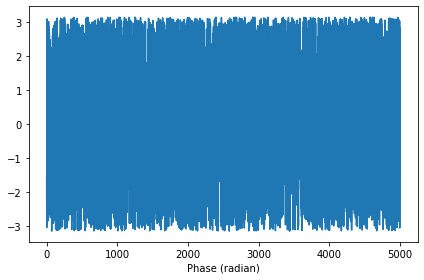Put the shuffled phases back into the spectrum. Each element in hs is a complex number with magitude $A$ and phase $\phi$, with which we can compute $A e^{i \phi}$

In :
i = complex(0, 1)
spectrum = wave.make_spectrum()
spectrum.hs = magnitude * np.exp(i * angle)


Convert the spectrum back to a wave (which uses irfft).

In :
wave2 = spectrum.make_wave()
wave2.normalize()
segment = wave2.segment(duration=0.005)
segment.plot()
decorate(xlabel='Time (s)')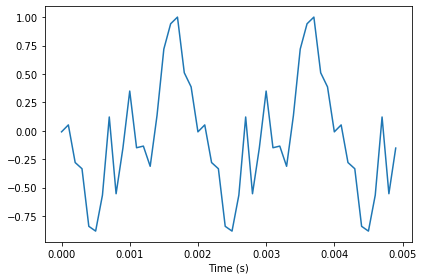Play the wave with the shuffled phases.

In :
wave2.make_audio()

Out:

For comparison, here's the original wave again.

In :
wave.make_audio()

Out:

Although the two signals have different waveforms, they have the same frequency components with the same amplitudes. They differ only in phase.

## Aliasing interaction¶

The following interaction explores the effect of aliasing on the harmonics of a sawtooth signal.

In :
def view_harmonics(freq, framerate):
"""Plot the spectrum of a sawtooth signal.

freq: frequency in Hz
framerate: in frames/second
"""
signal = SawtoothSignal(freq)
wave = signal.make_wave(duration=0.5, framerate=framerate)
spectrum = wave.make_spectrum()
spectrum.plot(color='C0')
decorate(xlabel='Frequency (Hz)', ylabel='Amplitude')
display(wave.make_audio())

In :
from ipywidgets import interact, interactive, fixed
import ipywidgets as widgets

slider1 = widgets.FloatSlider(min=100, max=10000, value=100, step=100)
slider2 = widgets.FloatSlider(min=5000, max=40000, value=10000, step=1000)
interact(view_harmonics, freq=slider1, framerate=slider2);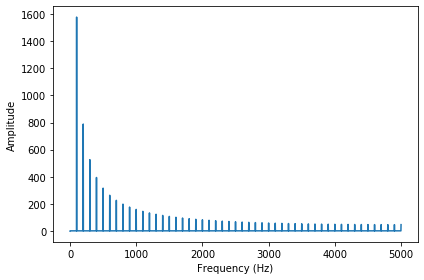In [ ]:


In [ ]: